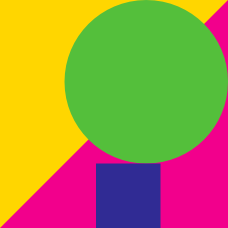Geometry

# Geometry Warmups: Level 3 Challenges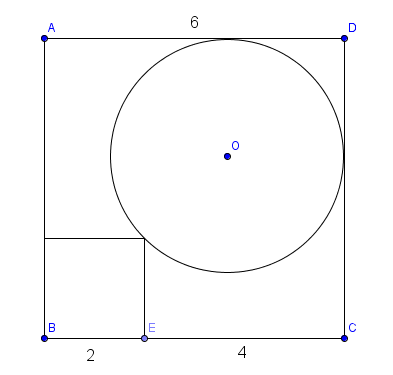In the figure above, we have a square and a circle inside a larger square.

Find the radius of the circle, to 3 decimal places.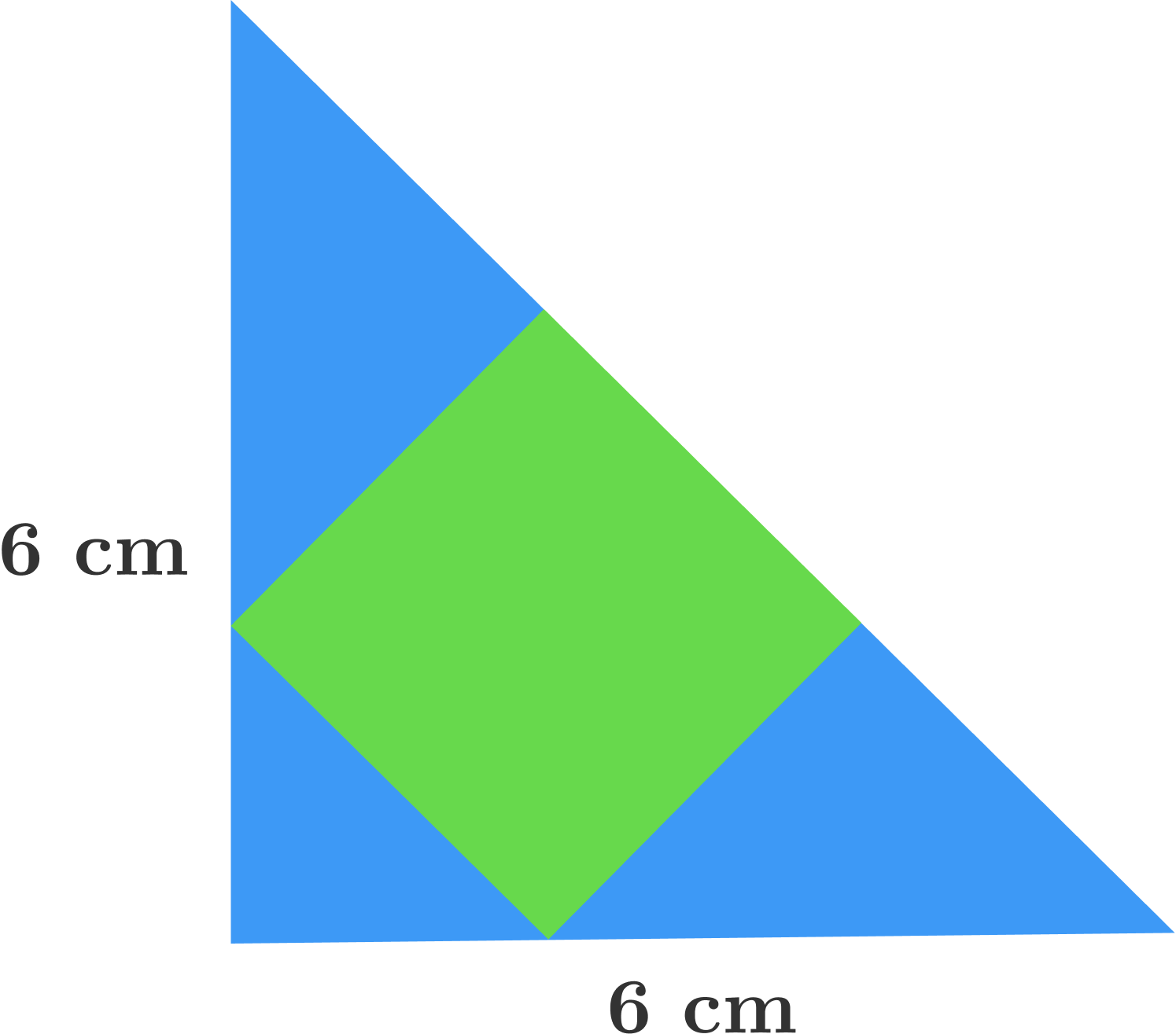Find the area (in $\text{cm}^2$) of the shaded green square in the blue isosceles right triangle, whose legs have length 6 cm.

$\tan6^{\circ} \times \tan42^{\circ} \times \tan66^{\circ} \times \tan78^{\circ}=\, ?$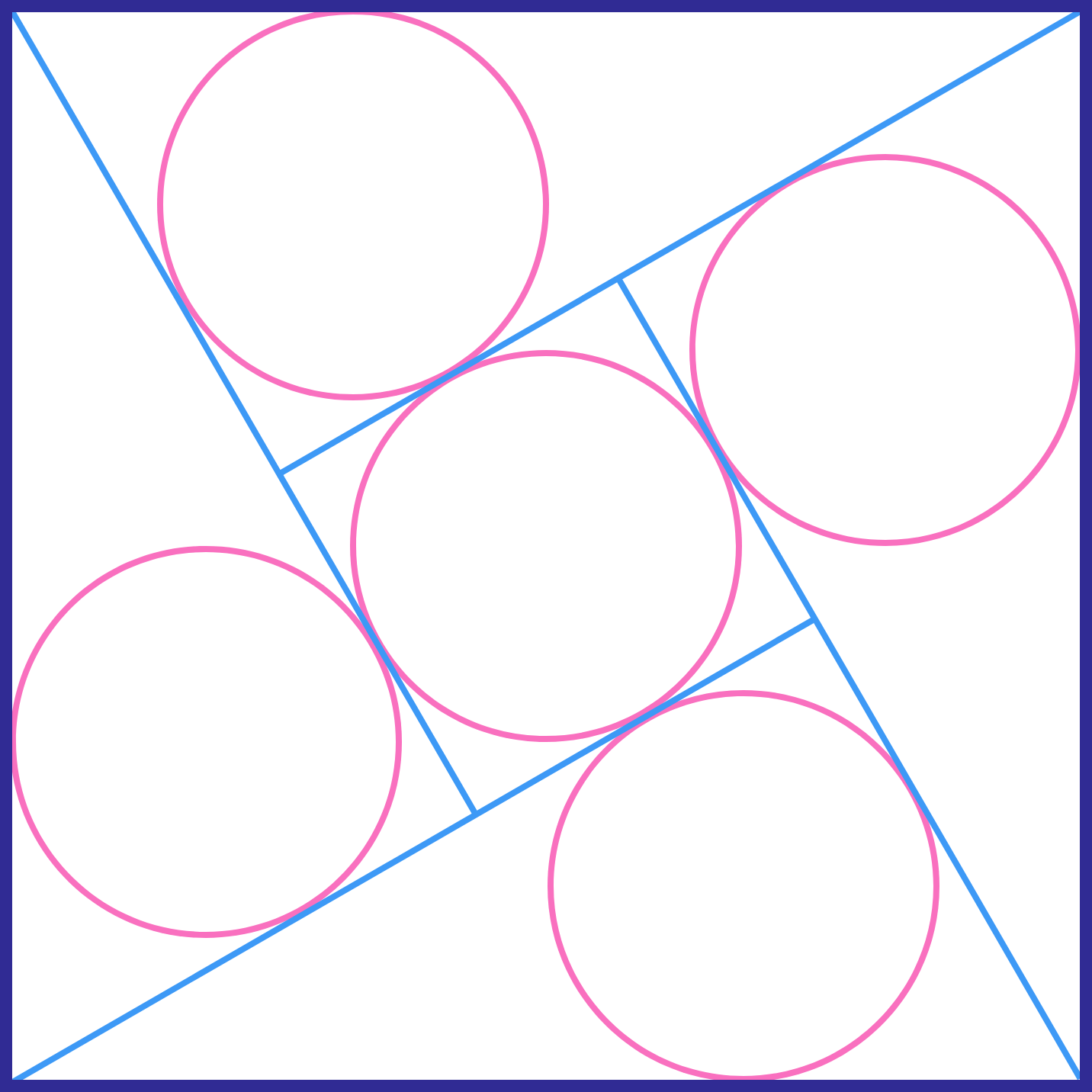The 5 circles have the same size. If the side of the large square is 1, what is the radius of each circle (to 3 decimal places)?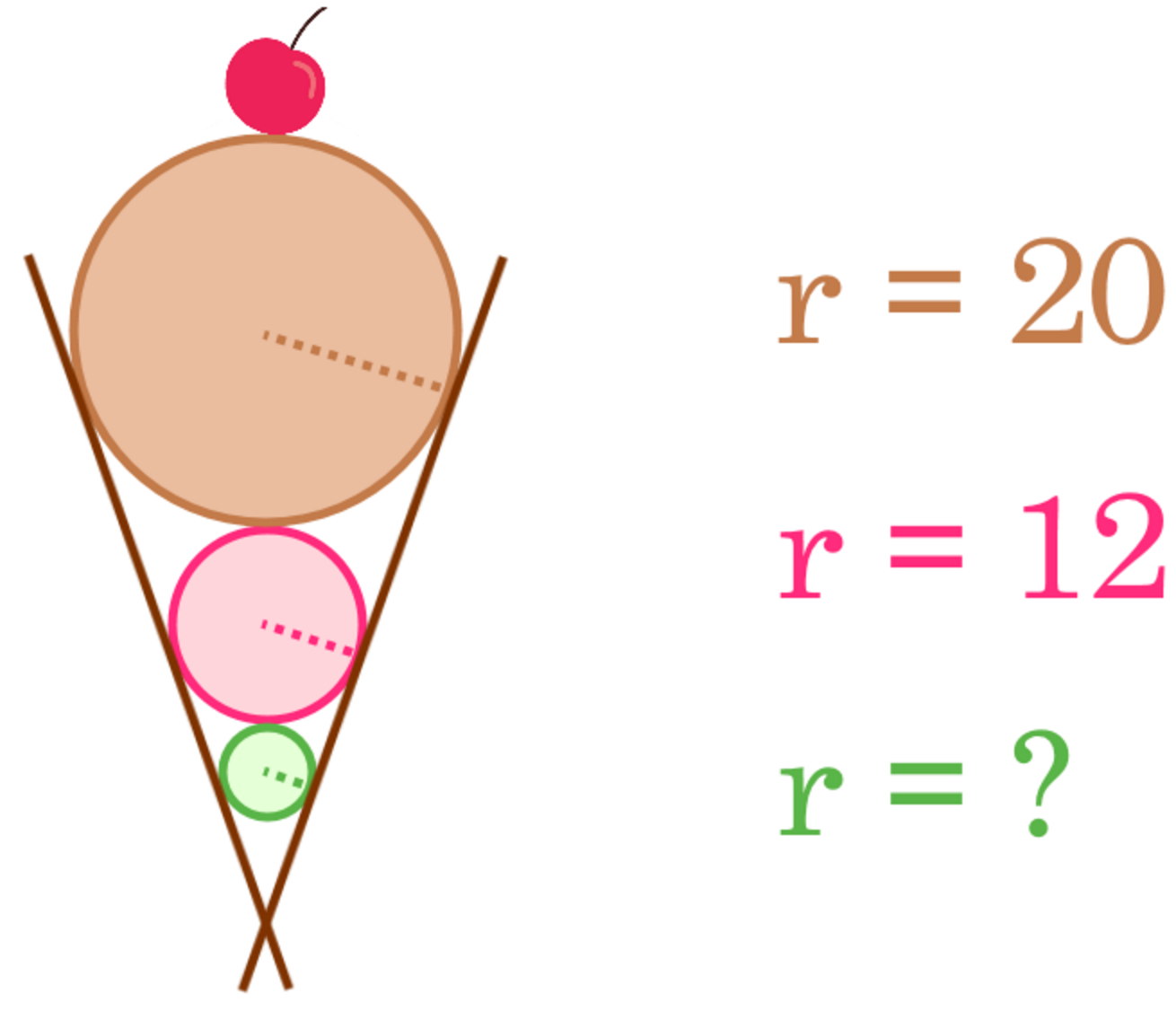Three balls are placed inside a cone such that each ball is in contact with the edge of the cone and the next ball. If the radii of the balls are 20 cm, 12 cm, and $r$ cm from top to bottom, what is the value of $r$?

×